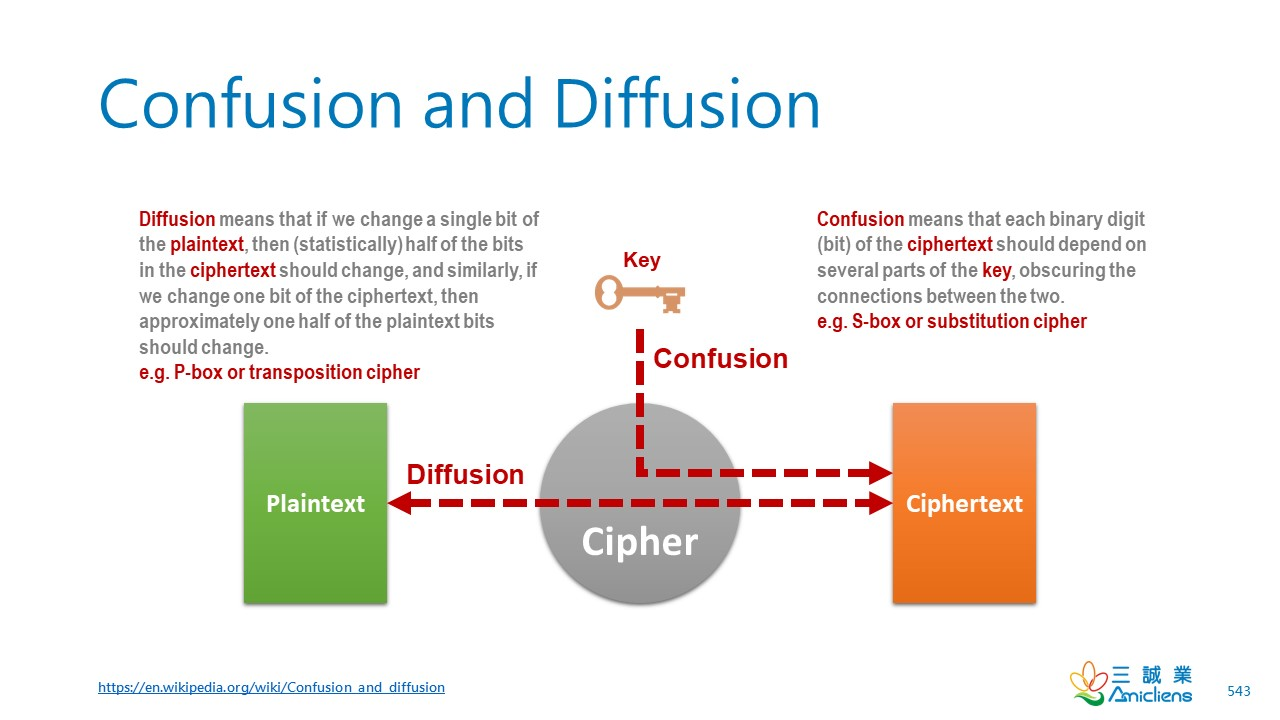# Confusion and Diffusion# A Mathematical Theory of Cryptography

In general, a statistical attack proceeds as follows. A certain statistic is measured on the intercepted cryptogram E. This statistic is such that for all reasonable M it assumes about the same value, SK, the value depending only on the particular key K that was used.

## Confusion

The method of confusion is to make the relation between the simple statistics of E and the simple description of K a very complex and involved one.

## Diffusion

In the method of diffusion the statistical structure of M which leads to its redundancy is “dissipated” into long range statistics — i.e., into statistical structure involving long combinations of letters in the cryptogram. The effect here is that the enemy must intercept a tremendous amount of material to tie down this structure, since the structure is evident only in blocks of very small individual probability. Furthermore even when he has sufficient material, the analytical work required is much greater since the redundancy has been diffused over a large number of individual statistics.

## Conclusion

The conclusion here is that for a good ciphering system steps should be taken either to diffuse or confuse the redundancy (or both).

Claude Elwood Shannon (April 30, 1916 – February 24, 2001)

# Confusion and Diffusion

In cryptography, confusion and diffusion are two properties of the operation of a secure cipher identified by Claude Shannon in his 1945 classified report A Mathematical Theory of Cryptography. These properties, when present, work to thwart the application of statistics and other methods of cryptanalysis.

https://en.wikipedia.org/wiki/Confusion_and_diffusion

# Product Cipher

In cryptography, a product cipher combines two or more transformations in a manner intending that the resulting cipher is more secure than the individual components to make it resistant to cryptanalysis. The product cipher combines a sequence of simple transformations such as substitution (S-box), permutation (P-box), and modular arithmetic. The concept of product ciphers is due to Claude Shannon, who presented the idea in his foundational paper, Communication Theory of Secrecy Systems.

https://en.wikipedia.org/wiki/Product_cipher

# Avalanche Effect

In the case of high-quality block ciphers, such a small change in either the key or the plaintext should cause a drastic change in the ciphertext.

The actual term was first used by Horst Feistel, although the concept dates back to at least Shannon’s diffusion.

https://en.wikipedia.org/wiki/Avalanche_effect